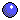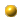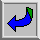## Zeno’s Paradox of the Race Course

Zeno argues that it is impossible for a runner to traverse a race course. His reason is that

“there is no motion, because that which is moving must reach the midpoint before the end” (6=A25, Aristotle, Physics 239b11-13).

Why is this a problem? Because the same argument can be made about half of the race course: it can be divided in half in the same way that the entire race course can be divided in half. And so can the half of the half of the half, and so on, ad infinitum.

So a crucial assumption that Zeno makes is that of infinite divisibility: the distance from the starting point (S) to the goal (G) can be divided into an infinite number of parts.

2. #### Progressive vs. Regressive versions

How did Zeno mean to divide the race course? That is, which half of the race course Zeno mean to be dividing in half? Was he saying (a) that before you reach G, you must reach the point halfway from the halfway point to G? This is the progressive version of the argument: the subdivisions are made on the right-hand side, the goal side, of the race-course.

Or was he saying (b) that before you reach the halfway point, you must reach the point halfway from S to the halfway point? This is the regressive version of the argument: the subdivisions are made on the left-hand side, the starting point side, of the race-course.

If he meant (a), the progressive version, then he was arguing that the runner could not finish the race. If he meant (b), the regressive version, then he was arguing that the runner could not even start the race. Either conclusion is repugnant to reason and common sense, and it seems impossible to ascertain which version Zeno had in mind.

But it turns out that it really doesn’t matter which version Zeno had in mind. For although this may not be obvious, the conclusions of the two versions of the argument are equivalent. Let us see why.

Since Zeno was generalizing about all motion, his conclusion was either (a) that no motion could be completed or (b) that no motion could be begun. But in order to begin a motion, one has to complete a smaller motion that is a part of it. For consider any motion, m, and suppose that m has been begun. It follows that some smaller initial portion of m has been completed; for if no such part of m has been completed, m could not have yet begun. Hence, if no motion can be completed, then none can be begun.

It is even more obvious that if no motion can be begun, then none can be completed. So the conclusion of (a) (“no motion can be completed”) entails, and is entailed by, the conclusion of (b) (“no motion can be begun”). That is, the two conclusions are logically equivalent. Hence we needn’t worry about how Zeno wanted to place the halfway points.

3. #### Terminology

 R the runner S the starting point (= Z0) G the end point Z1 the point halfway between S and G Z2 the point halfway between Z1 and G Zn the point halfway between Zn-1 and G Z-run a run that takes the runner from one Z-point to the next Z-point

4. #### Zeno’s Argument formulated

1. In order to get from S to G, R must make infinitely many Z-runs.

2. It is impossible for R to make infinitely many Z-runs.

3. Therefore, it is impossible for R to reach G.

5. #### Evaluating the argument

1. Is it valid? Yes: the conclusion follows from the premises.

2. Is it sound? I.e., is it a valid argument with true premises? This is what is at issue.

3. One might try to object to the first premise, (1), on the grounds that one can get from S to G by making one run, or two (from S to Z1 and from Z1 to G). But this is not an adequate response. For according to the definitions above, the runner, if he passes from S to G, will have passed through all the Z-points. But to do that is to make all the Z-runs.

Alternatively, one might object to (1) on the grounds that passing through all the Z-points (even though there are infinitely many of them) does not constitute making an infinite number of Z-runs. The reason might be that after you keep halving and halving the distance, you eventually get to distances that are so small that they are no larger than points. But points have no dimension, so no “run” is needed to “cross” one. But this is a mistake. For every Z-run, no matter how tiny, covers a finite distance (>0). No Z-run is as small as a point.

So we have established that the first premise is true. (Note: this does not establish that R can actually get from S to G. It only establishes that if he does, he will make all the Z-runs.)

4. The crucial premise is (2). Why can’t R make infinitely many Z-runs? Our difficulty here is that Zeno gives no explicit argument in support of (2).

6. #### Supporting the second premise

There are three possible reasons that might be given in support of (2).

1. To make all the Z-runs R would have to run infinitely far.

2. To make all the Z-runs R would have to run forever (i.e., for an infinite length of time).

3. To make all the Z-runs R would have to do something it is logically impossible to do. (I.e., the claim that R makes all the Z-runs leads to a logical contradiction.)

Which of these reasons did Zeno have in mind? Aristotle assumed that (b) was what Zeno intended (and he based his refutation on that assumption). More recent critics have suggested that Zeno’s argument can be made much more interesting if we use (c) to support his second premise. We will consider both (b) and (c) later. But since there is some reason to think that Zeno believed (a), we will begin there.

To see why one might think that Zeno had (a) in mind, we will examine a related argument that he actually gave: his argument against plurality. We will then return to the race course.Go to next lecture on Zeno against pluralityGo to previous lecture on Parmenides, Stage 2Return to the PHIL 320 Home Page